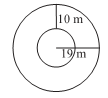# Ex.11.3 Q15 Perimeter and Area - NCERT Maths Class 7

Go back to  'Ex.11.3'

## Question

Find the circumference of the inner and the outer circles, shown in the adjoining figure? (Take $$π = 3.14$$)Video Solution
Perimeter And Area
Ex 11.3 | Question 15

## Text Solution

What is known?

Inner and outer circles of radius $$10 \rm\,m$$ and 1$$9 \rm\,m$$.

What is unknown?

The circumference of the inner and the outer circles.

Reasoning:

This question is straight forward, use the given information as the inner and outer radius of the circle is given $$10 \rm\,m$$ and 1$$9 \rm\,m$$, by using formula of the circumference of the circle you can find out the circumference of the inner and outer circles.

Steps:

Radius of the outer circle $$(r_1) = 19 \rm\,m$$

Therefore,

Circumference of outer circle

\begin{align}&= 2\pi {r_1} \\&= 2 \times 3.14 \times 19\\ &= 119.32{\rm{ }}\rm\,m\end{align}

Radius of inner circle$$(r_2)$$

\begin{align} &= 19 \,– 10 \\&= 9\rm\, m\end{align}

Therefore,

Circumference of outer circle

\begin{align} &= 2\pi {r_2}\\ &= 2 \times 3.14 \times 9\\ &= 56.52{\rm{ }}\rm \,m\end{align}

Therefore, the circumferences of inner and outer circles are $$56.52 \rm\,m$$ and $$119.32 \rm\,m$$ respectively.

Learn from the best math teachers and top your exams

• Live one on one classroom and doubt clearing
• Practice worksheets in and after class for conceptual clarity
• Personalized curriculum to keep up with school